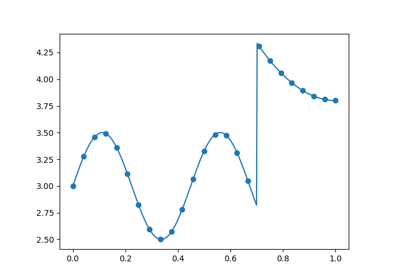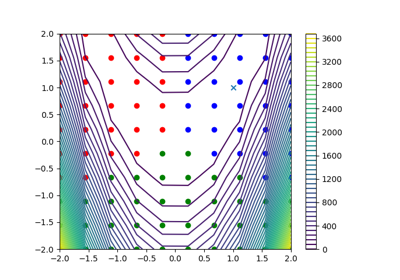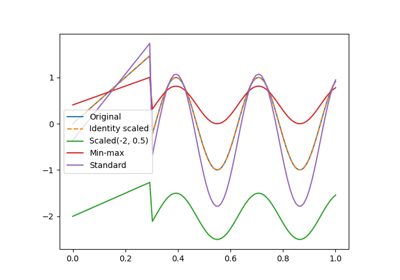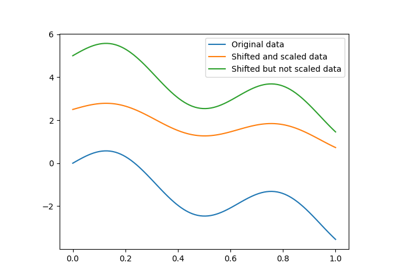gemseo / mlearning / transform / scaler

# scaler module¶

Scaling a variable with a linear transformation.

The Scaler class implements the default scaling method applying to some parameter $$z$$:

$\bar{z} := \text{offset} + \text{coefficient}\times z$

where $$\bar{z}$$ is the scaled version of $$z$$. This scaling method is a linear transformation parameterized by an offset and a coefficient.

In this default scaling method, the offset is equal to 0 and the coefficient is equal to 1. Consequently, the scaling operation is the identity: $$\bar{z}=z$$. This method has to be overloaded.

class gemseo.mlearning.transform.scaler.scaler.Scaler(name='Scaler', offset=0.0, coefficient=1.0)[source]

Bases: Transformer

Data scaler.

Parameters:
• name (str) –

A name for this transformer.

By default it is set to “Scaler”.

• offset (float) –

The offset of the linear transformation.

By default it is set to 0.0.

• coefficient (float) –

The coefficient of the linear transformation.

By default it is set to 1.0.

compute_jacobian(data)[source]

Compute Jacobian of transformer.transform().

Parameters:

data (ndarray) – The data where the Jacobian is to be computed.

Returns:

The Jacobian matrix.

Return type:

ndarray

compute_jacobian_inverse(data)[source]

Compute Jacobian of the transformer.inverse_transform().

Parameters:

data (ndarray) – The data where the Jacobian is to be computed.

Returns:

The Jacobian matrix.

Return type:

ndarray

duplicate()

Duplicate the current object.

Returns:

A deepcopy of the current instance.

Return type:

Transformer

fit(data, *args)

Fit the transformer to the data.

Parameters:
Return type:

NoReturn

fit_transform(data, *args)

Fit the transformer to the data and transform the data.

Parameters:
Returns:

The transformed data.

Return type:

ndarray

inverse_transform(data)[source]

Perform an inverse transform on the data.

Parameters:

data (ndarray) – The data to be inverse transformed.

Returns:

The inverse transformed data.

Return type:

ndarray

transform(data)[source]

Transform the data.

Parameters:

data (ndarray) – The data to be transformed.

Returns:

The transformed data.

Return type:

ndarray

CROSSED = False
property coefficient: float

The scaling coefficient.

name: str

The name of the transformer.

property offset: float

The scaling offset.

parameters: str

The parameters of the transformer.

## Examples using Scaler¶Quality measure for surrogate model comparison

Quality measure for surrogate model comparisonMixture of experts

Mixture of expertsScaler example

Scaler exampleTransformer pipeline example

Transformer pipeline example